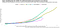# On The Double

## How long does a quantity take to double?

Our World in Data and news articles refer to the doubling time of COVID-19 confirmed cases and deaths.

This article looks at what doubling times are, and how to interpret this figure.

# Doubling Times

A doubling time is the time it takes for a quantity to double in size, assuming constant growth. Researchers often calculate this figure for population growth, whether cells, animals, or humans.

To use an example, imagine there were 1,000 rabbits on a island. Their population increases by 20% every year.

How long does it take for the number of rabbits on the island to double?

After one year, there are 1,200 rabbits. We then increase that number by 20%, to get 1,440 in the second year. By the third year, that number of rabbits is 1,728. After four years, that number is 2,074. I rounded that final figure, as our rabbit population should always be a whole number.

It takes around four years for the number of rabbits to double. To be more precise, the doubling time for our rabbit population is about 3.8 years.

# Cases and deaths

The Our World in Data page says the doubling time for COVID-19 confirmed deaths in Cameroon is five days. What does this mean?

On April 24th, the number of confirmed deaths was 49. Five days earlier, on April 19th, that number was 21. That was less than half of current total deaths. The page reports confirmed deaths doubled in five days.

Generally, it is useful to look at percentage growth rates. Sometimes, doubling times may give a better sense of how fast a quantity is growing.

We can calculate doubling times for confirmed deaths in countries. I use doubling time calculated over the previous seven days. This was to overcome weekly cycles in reporting deaths.Doubling times suggest slowing relative growth in deaths. (Image: ggplot2)

The higher the doubling time, the lower the underlying growth.

If the growth is exponential, the doubling time remains constant. That means the value is increasing by a fixed percentage each time. This is like the example rabbit population, increasing by 20% each year.

# Considerations

There are some things to consider when reading doubling times.

This measure is most appropriate when the underlying growth is exponential.

The doubling time calculation assumes that growth is constant between the two measurements. It also depends on how far apart in time you take the two measurements. In my graph, I used seven days. The graph would look different if I used one day intervals, or two weeks.

If there is no increase in the quantity, then the doubling time is not defined.

Doubling times can be an intuitive measure in the early stages of an epidemic.

The R code for the graph is available on GitHub. There is also an R Markdown page to view. Confirmed death by country comes from an Our World in Data CSV. The underlying data draws from the European Centre for Disease Prevention and Control.

This blog looks at the use of statistics in Britain and beyond. It is written by RSS Statistical Ambassador and Chartered Statistician @anthonybmasters.

## More from Anthony B. Masters

This blog looks at the use of statistics in Britain and beyond. It is written by RSS Statistical Ambassador and Chartered Statistician @anthonybmasters.